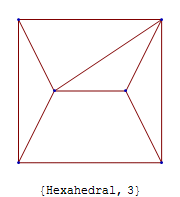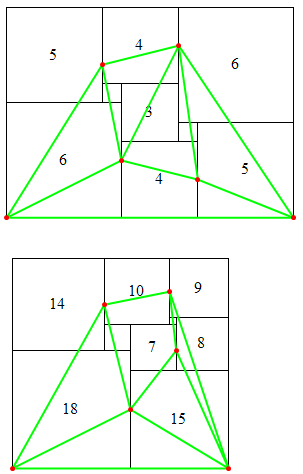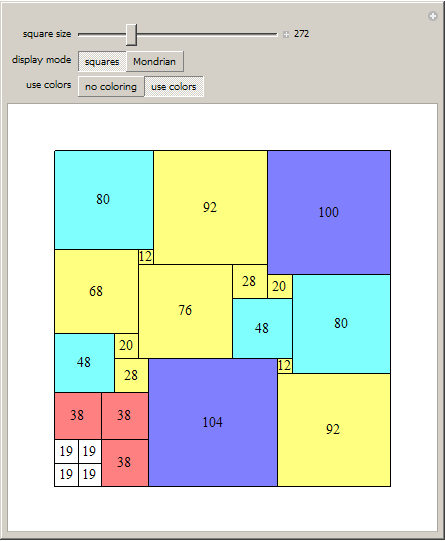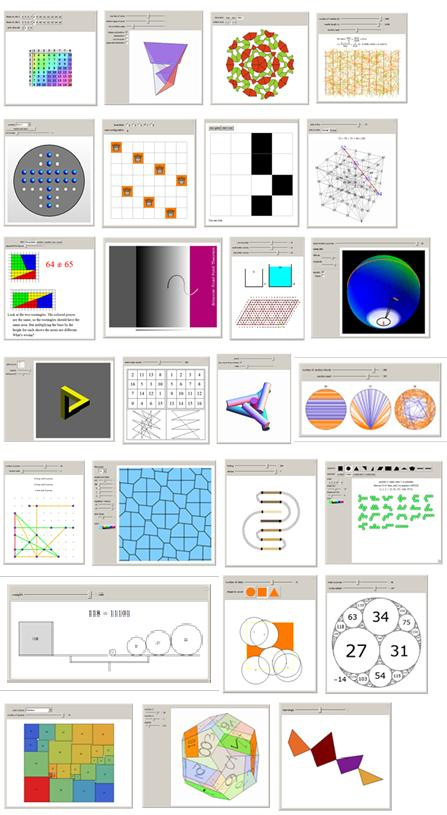Browse by Topic
Related Topics

# Remembering Martin Gardner

In Winning Ways for Your Mathematical Plays, noted mathematicians Elwyn Berlekamp, John Conway, and Richard Guy have this dedication: “To Martin Gardner, who has brought more mathematics to more millions than anyone else.”

Martin Gardner passed away on May 22, 2010, and I talk about my own introduction to his work at the Wolfram|Alpha Blog. On May 21, I was asked for the best book about math for a young adult. I suggested Martin Gardner’s Mathematical Games (CD-ROM), which contains his 15-book canon. In my opinion, it’s still the best book series for sparking an interest in mathematics.

In a way, Wolfram Research has been trying to beat Martin in the noble goal of bringing math and science to the millions. We’ve partnered with CBS/Paramount for the NUMB3RS TV show, our CEO Stephen Wolfram wrote A New Kind of Science, co-founder Theodore Gray made what I think is the best periodic table in the world, and we built MathWorld, the most comprehensive math encyclopedia on the web.

And of course, there’s Mathematica. Gardner’s The Second Scientific American Book of Mathematical Puzzles and Diversions includes a rare guest column by mathematician William Tutte, in which he relates the story of himself, C. A. B. Smith, A. H. Stone, and R. L. Brooks. In 1936, they knew that a 32×33 rectangle could be divided into 9 squares of different sizes. Such a thing was called a perfect rectangle. They wondered if they could find a perfect square, and set about cataloging perfect rectangles. They noticed that their “Smith diagram” notation was equivalent to a graph, and then realized that electrical network theory could convert an arbitrary polyhedral graph into a squared rectangle.

Mathematica can do that. For example, the graph can be one of the hexahedral graphs.The content of this paragraph is pretty complicated. In fact, it took me weeks to understand the next few sentences, and I’ve studied graph theory for years. (That is part of why I treasure of Martin’s writings: I know where something difficult is explained.) From the graph above, a row of the incidence matrix is deleted to give ground (ground reference node). Multiplying ground by its transpose gives the Kirchhoff matrix (K, also called the Laplacian), whose determinant (det) counts spanning trees. The edge current matrix (edge) is the product of the ground transpose, the volt matrix (volt = det K), and ground. Dividing each row by the greatest common divisor gives a matrix of final edge currents. Each row is then a solution for how squares can be placed according to the original graph. For example, with the graph above, here are two of the solutions given by this method:Each vertical line in the diagram is equivalent to one of the nodes of the original graph, and each square corresponds to one of the edges. From there, finding all the solutions of a given order is as simple as running the program on all the polyhedral graphs of that order.

In Mathematical Carnival, Martin discusses the Mrs. Perkins’s Quilt problem—divide a 13×13 square into the fewest possible number of smaller squares. Over the past few years, Martin has been updating all his books, and I’ve been working with Richard Guy and Stuart Anderson to update this particular chapter. Analyzing tens of millions of planar graphs with Mathematica and the methods shown above has yielded many improvements to known solutions, most of which are shown within this Wolfram Demonstration:The above is just one of over 6,000 Demonstrations, many of which tie in directly with Martin’s work. I list some of them below—many of these were written by Gardner’s original correspondents. The Wolfram Demonstrations Project fits in with Martin’s goal, and our goal, to make math interesting for as many people as possible.